Related Articles

# Matplotlib.pyplot.legend() in Python

• Difficulty Level : Easy
• Last Updated : 12 Apr, 2020

Matplotlib is one of the most popular Python packages used for data visualization. It is a cross-platform library for making 2D plots from data in arrays. Pyplot is a collection of command style functions that make matplotlib work like MATLAB. Each pyplot function makes some change to a figure: e.g., creates a figure, creates a plotting area in a figure, plots some lines in a plotting area, decorates the plot with labels, etc.

## Matplotlib.pyplot.legend()

A legend is an area describing the elements of the graph. In the matplotlib library, there’s a function called legend() which is used to Place a legend on the axes.

The attribute Loc in `legend()` is used to specify the location of the legend.Default value of loc is loc=”best” (upper left). The strings ‘upper left’, ‘upper right’, ‘lower left’, ‘lower right’ place the legend at the corresponding corner of the axes/figure.

The attribute bbox_to_anchor=(x, y) of legend() function is used to specify the coordinates of the legend, and the attribute ncol represents the number of columns that the legend has.It’s default value is 1.

Syntax:

matplotlib.pyplot.legend([“blue”, “green”], bbox_to_anchor=(0.75, 1.15), ncol=2)

The Following are some more attributes of function `legend()` :

• shadow: [None or bool] Whether to draw a shadow behind the legend.It’s Default value is None.
• markerscale: [None or int or float] The relative size of legend markers compared with the originally drawn ones.The Default is None.
• numpoints: [None or int] The number of marker points in the legend when creating a legend entry for a Line2D (line).The Default is None.
• fontsize: The font size of the legend.If the value is numeric the size will be the absolute font size in points.
• facecolor: [None or “inherit” or color] The legend’s background color.
• edgecolor: [None or “inherit” or color] The legend’s background patch edge color.

#### Ways to use legend() function in Python –

Example 1:

 `import` `numpy as np``import` `matplotlib.pyplot as plt`` ` `# X-axis values``x ``=` `[``1``, ``2``, ``3``, ``4``, ``5``]`` ` `# Y-axis values ``y ``=` `[``1``, ``4``, ``9``, ``16``, ``25``]`` ` `# Function to plot  ``plt.plot(x, y)`` ` `# Function add a legend  ``plt.legend([``'single element'``])`` ` `# function to show the plot``plt.show()`

Output :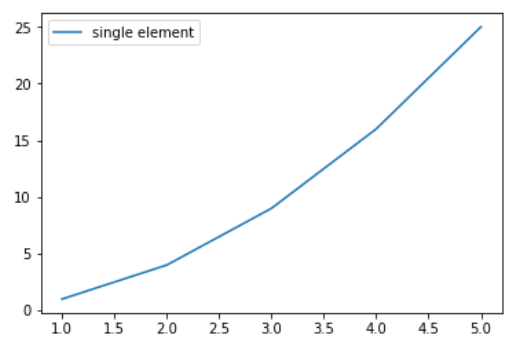Example 2:

 `# importing modules``import` `numpy as np``import` `matplotlib.pyplot as plt`` ` `# Y-axis values``y1 ``=` `[``2``, ``3``, ``4.5``]`` ` `# Y-axis values ``y2 ``=` `[``1``, ``1.5``, ``5``]`` ` `# Function to plot  ``plt.plot(y1)``plt.plot(y2)`` ` `# Function add a legend  ``plt.legend([``"blue"``, ``"green"``], loc ``=``"lower right"``)`` ` `# function to show the plot``plt.show()`

Output :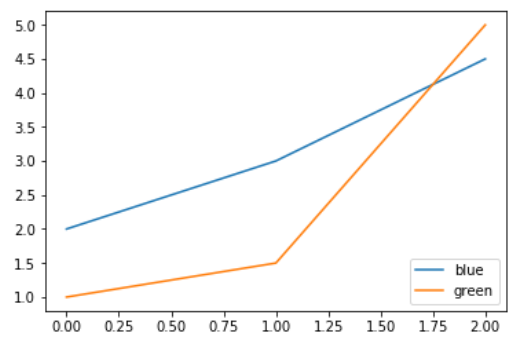Example 3:

 `import` `numpy as np``import` `matplotlib.pyplot as plt`` ` `# X-axis values``x ``=` `np.arange(``5``)`` ` `# Y-axis values``y1 ``=` `[``1``, ``2``, ``3``, ``4``, ``5``]`` ` `# Y-axis values ``y2 ``=` `[``1``, ``4``, ``9``, ``16``, ``25``]`` ` `# Function to plot  ``plt.plot(x, y1, label ``=``'Numbers'``)``plt.plot(x, y2, label ``=``'Square of numbers'``)`` ` `# Function add a legend  ``plt.legend()`` ` `# function to show the plot``plt.show()`

Output :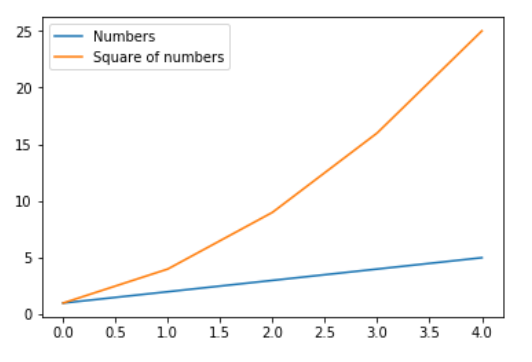Example 4:

 `import` `numpy as np``import` `matplotlib.pyplot as plt`` ` `x ``=` `np.linspace(``0``, ``10``, ``1000``)``fig, ax ``=` `plt.subplots()`` ` `ax.plot(x, np.sin(x), ``'--b'``, label ``=``'Sine'``)``ax.plot(x, np.cos(x), c ``=``'r'``, label ``=``'Cosine'``)``ax.axis(``'equal'``)`` ` `leg ``=` `ax.legend(loc ``=``"lower left"``);`

Output: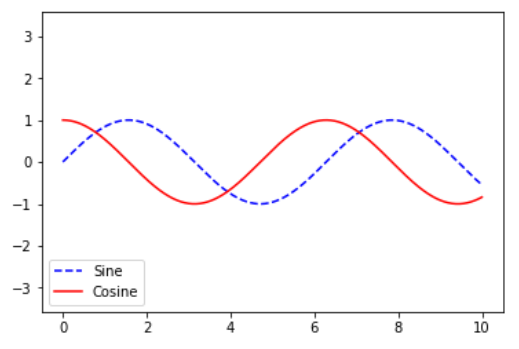Example 5:

 `# importing modules``import` `numpy as np``import` `matplotlib.pyplot as plt``  ` `# X-axis values``x ``=` `[``0``, ``1``, ``2``, ``3``, ``4``, ``5``, ``6``, ``7``, ``8``]``  ` `# Y-axis values``y1 ``=` `[``0``, ``3``, ``6``, ``9``, ``12``, ``15``, ``18``, ``21``, ``24``]``# Y-axis values ``y2 ``=` `[``0``, ``1``, ``2``, ``3``, ``4``, ``5``, ``6``, ``7``, ``8``]``  ` `# Function to plot  ``plt.plot(y1, label ``=``"y = x"``)``plt.plot(y2, label ``=``"y = 3x"``)``  ` `# Function add a legend  ``plt.legend(bbox_to_anchor ``=``(``0.75``, ``1.15``), ncol ``=` `2``)``  ` `# function to show the plot``plt.show()`

Output: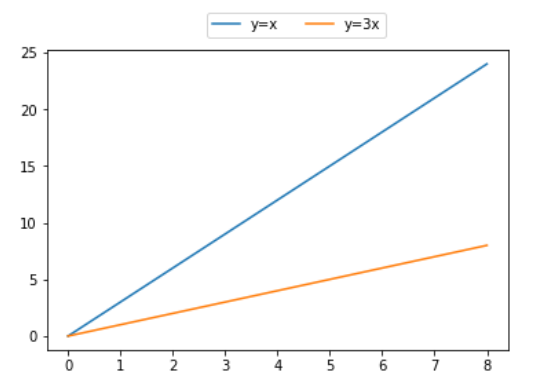Attention geek! Strengthen your foundations with the Python Programming Foundation Course and learn the basics.

To begin with, your interview preparations Enhance your Data Structures concepts with the Python DS Course. And to begin with your Machine Learning Journey, join the Machine Learning – Basic Level Course

My Personal Notes arrow_drop_up